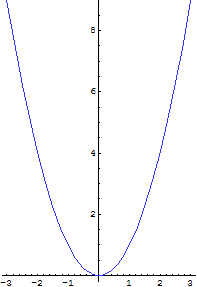abstractmath.org 2.0
help with abstract math

Produced by Charles Wells     Revised 2017-04-13

Introduction to this website    website TOC    website index   blog



# UNDERSTANDING MATH

In mathematics you don’t understand things, you just get used to them. –John von Neumann

## Definitions

• One piece of information you must have about a math concept is its definition.
• Every proof of a fact about the concept must be based on a logical chain of reasoning starting with the definition.
• The definition is one path to understanding the concept but not the only one

## Math objects

• Mathematical objects are what mathematics is “about”.
• The number $42$ is a math object.
• The set of even positive integers is a math object.  Even though it is an infinite set, it is a single math object.
• The function $f(x):={{\sin }}x$ is a math object.  Its value can be computed at many different numbers but it is a single, static math object. You can visualize going along it from left to right, which makes you go up and down over and over between $1$ and $-1$, and it is useful to visualize it that way (see Images and Metaphors), but it is a single, static math object.
• We talk and think about math objects in some of the same ways that we talk about physical objects, but in other ways they are not like physical objects.

## Math Structures

A mathematical structure is a special kind of math object defined as a set with some associated objects called structure.  Equivalence relations, partitions, groups and topological spaces are examples of mathematical structures.

## Representations and models

“Representation” and “model” have several related meanings.

• We can represent the number $42$ in binary notation as $101010$ and in hex as $2A$.
• We can represent a function by its formula or by its graph, or sometimes by a listing of its values.
• When we think about some math objects we may have a mental representation of them (like whooshing up and down on the graph of the sine function).
• We can model the trajectory of a ball by a function.
• A group is a model

## Images and metaphors• We think of a function that has a positive derivative as “going up”.  But the function isn’t really going up, it just sits there like your pet rock.
• We think of the parabola $y={{x}^{2}}$ as like a bowl whose sides go up forever.  But “forever” is a metaphor.  There is no time involved.
• Besides, we may forget that the sides of the parabola go out in the positive and negative x­ direction “forever”, too (every vertical line cuts the graph!), even though the picture looks like they are too steep to do that.

As these examples illustrate, we think about math objects in terms of images and metaphors that we have developed out of our experience with them.  These are valuable insights but they generally cannot be used to prove theorems about them.

There is a way of thinking about any math object that helps when you are trying to prove something: the rigorous view of math objects, also discussed in this chapter.

## Conceptual and Computational

When mathematicians consider a math object they are typically interested in two different aspects of the object:

• What is it? What properties does it have?  How is it different from other math objects?  I want a conceptual understanding of the object.
• How do I compute with it?  How do I find a value of the object (if that makes sense)?  How to I tell how big it is (in some sense of big)?  How do I determine in an efficient way what properties it has?

Proofs can have a conceptual side and a computational side too.

• A conceptual proof helps you understand why the statement is true.
• A computational proof may be easier to check systematically to see if it is correct, and to automate using some suitable computer program.

## Other aspects of understanding math

This catch-all chapter talks about several special phenomena that are involved in understanding math.